Next: Example: Up: An algebraic characterization of Previous: LP's in ``standard form''

#### Basic Feasible Solutions: An algebraic characterization of extreme points for LP's in ``standard form''

For LP's in ``standard form'' the previous characterization of extreme points as the solution of n linearly independent binding constraints, which is, furthermore, a feasible point, can become even more concise. Consider, for instance, the LP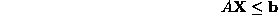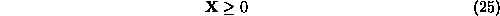with m technological constraints and n variables. In ``standard form'', it becomes: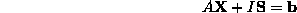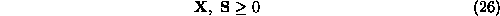where I is the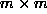identity matrix, and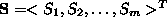is the vector of slack variables. It is interesting to notice that for every binding constraint from the m+n constraints of the original formulation (Eq. 25), one of the m+n variables of the ``standard form'' formulation must be equal to 0. Specifically, if the i-th technological constraint is binding, the corresponding slack variable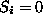. Similarly, if one of the sign restriction constraints is binding, then the corresponding varialbe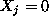. Since an extreme point of the feasible region of formulation 25 requires n binding constraints for its definition, it follows that n from the n+m variables in the corresponding solution of the ``standard form'' formulation (Eq. 26) must be equal to zero. Furthermore, since this point is uniquely defined, the system of equations defined by the m technological constraints in the ``standard form'' formulation and the m remaining variables, must have a unique solution. In other words, the columns of the ``standard form'' formulation corresponding to these m variables must be linearly independent (and their determinant must have a nonzero value). Finally, since the extreme point considered belongs in the feasible region of the problem, it follows that the unique solution of the aforementioned system of m equations in the m nonzero variables must be nonnegative (to meet the sign restrictions required by ``standard form'').

The structure of the ``standard form'' solutions corresponding to extreme points of the original feasible region (i.e., that defined with respect to the primary LP variables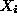), described for the example above, actually applies to any other LP in ``standard form''. We formally characterize this structure through the definition of basic feasible solutions for LP's in ``standard form'' (taken from Winston, ``Introduction to Mathematical Programming'').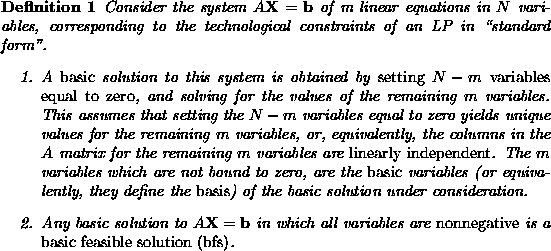Next: Example: Up: An algebraic characterization of Previous: LP's in ``standard form''

UAL Data
Fri Jun 20 15:03:05 CDT 1997